Support the Monkey! Tell All your Friends and TeachersHome MonkeyNotes Printable Notes Digital Library Study Guides Study Smart Parents Tips College Planning Test Prep Fun Zone Help / FAQ How to Cite New Title Request

19.2 Electrostatic Field

The mathematical form of Coulomb's law is similar to that of Newton's Law of gravitation. The gravitational force - at least in the Newtonian context - is called action-at-a-distance-force. The coulomb force may also be regarded as action-at-a-distance-force. However the action-at-a-distance-force has infinite speed of propagation and as we know that no signal can travel with infinite speed.

In electro-magnetism the force is treated as a force through a field. For static charges and steady currents there is no essential difference between the two view points, but when we have to deal with moving charges and non-steady currents, the action-at-a-distance-force becomes untenable and we must take recourse to action through field.

We define an Electrostatic field as the region of space in which a test charge (sufficiently small, so that it does not disturb the field) experiences electrostatic force, the mathematical expression of the force being the Coulomb's law expression. The Electrostatic field may or may not explicitly contain source charges within it.

Intensity of Electrostatic field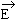The vectoris defined at any point in the given electrostatic field as the electrostatic force per unit positive test charge q placed at the given point in the field.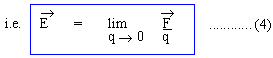S.I Unit of E is therefore 1 Newton / Coulomb.Your browser does not support the IFRAME tag.

Potential in electrostatic field V

The electrostatic field is a conservative field, i.e, its line integral along a closed curve is always zero.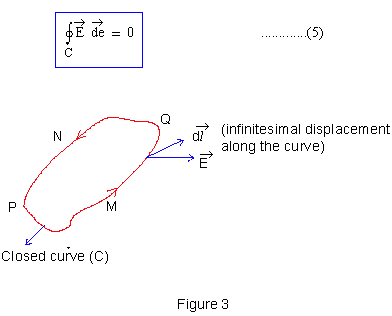The important Corollary of equation (5) is the work done per unit positive charge, in moving it from P to Q, is independent of the Path joining P to Q.

For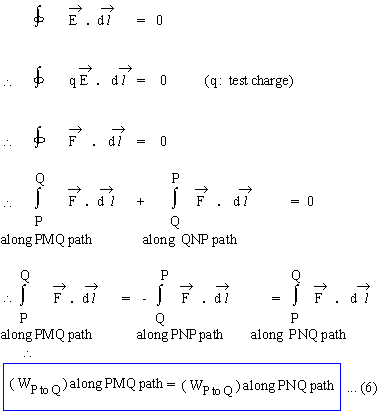\ WP to Q is independent of the path.

This property of an electrostatic field, can be used to define a scalar quantity V called the electrostatic potential, in the following way.

The negative of work done per unit positive test charge by the electrostatic force, in moving it from point P to Q, is defined to as the potential difference between the points Q and P.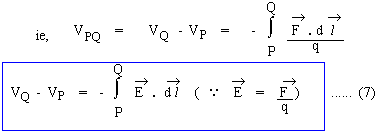If the point P is such that VP = 0 can be chosen, then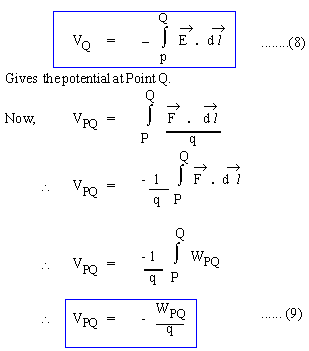S.I Unit of Potential or Potential Difference is therefore 1 Joule/ Coulomb, which is called 1 Volt, i.e, 1 Volt = 1 Joule / Coulomb.

Intensity and Potential in the field of a point source charge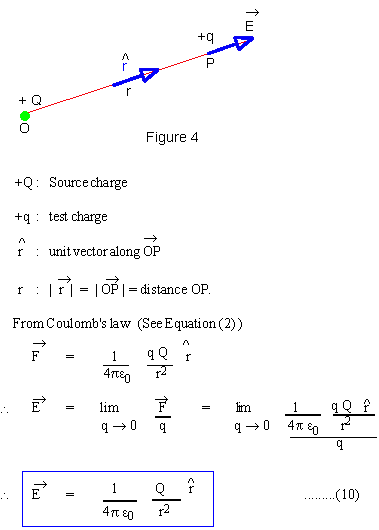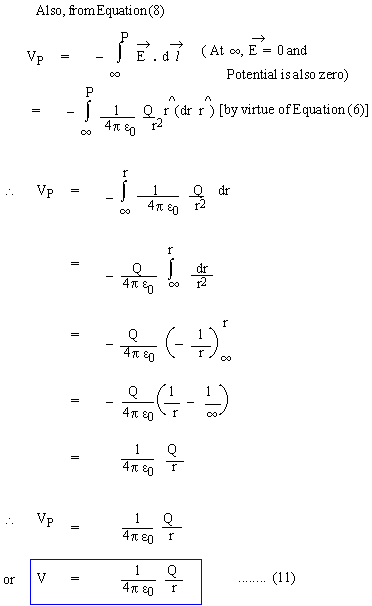Note : The customary negative sign in the definition of potential, renders it as a positive quantity in the field of positive source charge.

Equations (10) and (11) are closely related in the differential form, rather than the integral form of equation (8)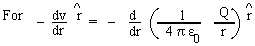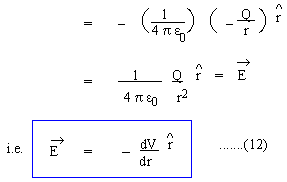Equation (12) expresses the relation betweenand V in the field of a point source charge, but it can be shown that it is a completely general result which may be expressed as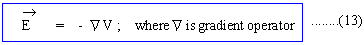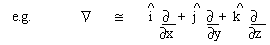is Cartesian co-ordinate system.

Field of a Discrete System

According to the superposition principle of forces, it is obvious that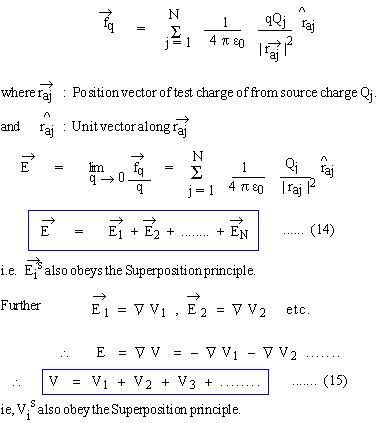Index

Electricity and Magnetism

19.1 Coulomb's Law
19.2 Electrostatic Field
19.3 Gauss's Law
19.4 CapacitorsSearch: All Products Books Popular Music Classical Music Video DVD Toys & Games Electronics Software Tools & Hardware Outdoor Living Kitchen & Housewares Camera & Photo Cell Phones Keywords: